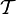# Congruence condition relating number of subgroups in maximal subgroups and number of subgroups in the whole group

Jump to: navigation, search

## Statement

Suppose$G$ is a group of prime power order. Suppose$\mathcal{S}$ is a collection of subgroups of$G$. For any subgroup$H$ of$G$, denote by$n(H)$ the number of subgroups of$H$ that are in$\mathcal{S}$. Then, if$G \notin \mathcal{S}$, we have:$n(G) \equiv \sum_{M \max G} n(M) \pmod p$

If$G \in \mathcal{S}$, we have:$n(G) \equiv 1 + \sum_{M \max G} n(M) \pmod p$.

## Facts used

1. Equivalence of definitions of maximal subgroup of group of prime power order (specifically, nilpotent implies every maximal subgroup is normal, and prime power order implies nilpotent, so all maximal subgroups are normal in groups of prime power order).
2. Fourth isomorphism theorem
3. Formula for number of maximal subgroups of group of prime power order

## Proof

Note that the$G \in \mathcal{S}$ case follows directly from the$G \notin \mathcal{S}$ case, so we restrict attention to the$G \notin \mathcal{S}$ case.

Given:$G$ is a group of prime power order.$\mathcal{S}$ is a collection of subgroups of$G$. For any subgroup$H$ of$G$, denote by$n(H)$ the number of subgroups of$H$ that are in$\mathcal{S}$.$G \notin \mathcal{S}$

To prove:$n(G) \equiv \sum_{M \max G} n(M) \pmod p$, where the summation is over maximal subgroups of$G$. Note that$n(G) = |\mathcal{S}|$.

Proof:

Step no. Assertion/construction Facts used Given data used Previous steps used Explanation
1 Let$\mathcal{T}$ be the set of ordered pairs$(H,M)$ where$H \in \mathcal{S}$ and$M$ is a maximal subgroup of$G$ containing$H$.
2$|\mathcal{T}| = \sum_{M \max G} n(M)$. Step (1) [SHOW MORE]
3$|\mathcal{T}|$ is the sum, over$H \in \mathcal{S}$, of the number of maximal subgroups of$G$ containing$S$. Step (1) Step-direct.
4 For any subgroup$H$ of$G$, the maximal subgroups of$G$ containing$H$ are precisely the same as the maximal subgroups of$G$ containing the normal closure$H^G$ of$H$ in$G$. Fact (1) [SHOW MORE]
5 For any subgroup$H$ of$G$, the maximal subgroups of$G$ containing$H$ correspond to maximal subgroups of$G/H^G$ where$H^G$ is the normal closure of$H$ in$G$. Fact (2) Step (4) Step-fact combination direct.
6 For any proper subgroup$H$ of$G$, the number of maximal subgroups of$G$ containing$H$ is congruent to 1 mod$p$. Fact (3) Steps (4), (5) [SHOW MORE]
7$|\mathcal{T}| \equiv |\mathcal{S}| \pmod p$. Note that$|\mathcal{S}| = n(G)$.$G \notin \mathcal{S}$ Steps (3), (6) [SHOW MORE]
8$n(G) \equiv \sum_{M \max G} n(M) \pmod p$ Steps (2), (7) Step-combination direct.